Computational & Technology Resources
an online resource for computational,
engineering & technology publications
Civil-Comp Proceedings
ISSN 1759-3433
CCP: 83
PROCEEDINGS OF THE EIGHTH INTERNATIONAL CONFERENCE ON COMPUTATIONAL STRUCTURES TECHNOLOGY
Edited by: B.H.V. Topping, G. Montero and R. Montenegro
Paper 278

Calculation of Eigenvalues of a Piezoelastic Beam with the Pseudospectral Method

M. Kekana

Centre for Advanced Materials, Manufacturing and Prototyping Research, Department of Mechanical Engineering, Durban Institute of Technology, South Africa

Full Bibliographic Reference for this paper
M. Kekana, "Calculation of Eigenvalues of a Piezoelastic Beam with the Pseudospectral Method", in B.H.V. Topping, G. Montero, R. Montenegro, (Editors), "Proceedings of the Eighth International Conference on Computational Structures Technology", Civil-Comp Press, Stirlingshire, UK, Paper 278, 2006. doi:10.4203/ccp.83.278
Keywords: Euler beam, eigenvalue problem, pseudospectral method, piezoelastic.

Summary
A number of mathematical models of piezoelastic structures (beams, plates and shells) derived using various theories have been reported. Two approaches in feedback control using piezoelectric sensor and actuator pair have been adopted. One assumes the measured signal to be the electric current, hence the closed circuit mode. The other assumes the measured signal to be the electric potential, hence the open circuit mode. This study is concerned with the latter approach; that is, the open circuit mode.

Analytical and approximate methods have been implemented with great success in solving the differential equation of motion of piezoelastic structures. These include the classical method , Fourier analysis , Green's method , finite element method , amongst others. However, the literature reviewed in the field of piezoelastic structures is devoid of the use of the pseudospectral method in solving the piezoelastic differential equations. Very few articles in solid mechanics use this method compared with those in fluid mechanics . This study contributes by solving the same using the psuedospectral method. The method is elegant, highly accurate and does not require mathematical rigour of the methods mentioned above.

The study considers the free vibration problem of a piezoelastic beam. The differential equation of motion is derived based on the Euler beam theory. The kinematic behaviour is represented by the variable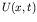, which represents the deflection of the neutral axis of the beam. The variable separable technique is used, i.e.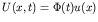, and a sinusoidal motion in time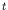is assumed for the temporal function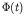. The spatial differential eigenvalue problem is then solved using the pseudospectral method. Following a simple procedure described below, the method is guaranteed to give an error which decreases exponentially fast with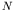, the number of degrees of freedom. The method first chooses a set of spectral basis functions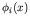that satisfy the boundary conditions. Next, it approximates the eigenfunction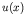by the-term truncation of the spectral series with unknown coefficients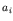. That is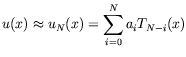(49)

where the basis functions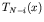's are the Chebyshev polynomials. These functions are suitable for this problem since the solution is non-symmetric. The approximate solution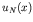is substituted into the differential equation. The method demands that the residual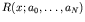of the eigenvalue problem be zero at the collocation points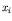, which are chosen here as the roots of the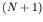th Chebyshev polynomial. The requirement results in the algebraic system of equations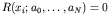, which can be recast in the standard eigenvalue matrix form. The final step is to call the library software to solve the matrix eigenvalue problem.

If the eigenmodes are needed, they may be evaluated by substituting the appropriate matrix eigenmode as the coefficients ofand then summing the series at each point of the graph. The-dimensional descretisation matrix of the differential equation usually haseigenvalues. Not all the matrix eigenvalues will be good approximations to those of the differential equation. Numerical results show that one typically obtains about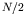matrix eigenvalues that are a close approximation of those of the differential equation.

With a bit of care, the pseudospectral method is a very robust and efficient method for solving linear differential eigenvalue problems. The exponential rate of convergence withimplies that it is possible to distinguish acceptable eigenvalues from unacceptable eigenvalues. Also, it is easy to program and check than the hand-derivation of the analytic type solution.

References
1
M. Kekana, P. Tabakov and M. Walker, "A shape control model for piezo-elastic structures based on divergence free electric displacement", International Journal of Solids and Structures, 40, 715-727, 12003. doi:10.1016/S0020-7683(02)00611-X
2
H.S. Tzou, Y. Bao and V.B. Venkayya, "Parametric study of segmented transducers laminated on cylindrical shells, part 1: Sensor patches", Journal of Sound and Vibration, 97(2), 207-224 1996. doi:10.1006/jsvi.1996.0526
3
S. Adali, I.S. Sadek, J.C. Bruch, Jr, J.M. Sloss, "Orthotropic piezoelectric patches for control of symmetric laminates via an integral equation", Composite Structures, 62, 271-277, 2003. doi:10.1016/j.compstruct.2003.09.025
4
M. Kekana, "Finite element modelling of laminated piezo-elastic structures: Lyapunov stability analysis", Journal of Sound and Vibration, 256(3), 463-473, 2002. doi:10.1006/jsvi.2002.5000
5
J.P. Boyd, "Chebyshev and Fourier spectral methods", 2edition, Dover Publications, 2000.

purchase the full-text of this paper (price £20)